# DAV Class 3 Maths Chapter 5 Brain Teasers Solutions

The DAV Class 3 Maths Book Solutions and DAV Class 3 Maths Chapter 5 Brain Teasers Solutions of Division offer comprehensive answers to textbook questions.

## DAV Class 3 Maths Ch 5 Brain Teasers Solutions

(Multiplication and Division)

Question 1.

(a) If Dividend = 18, Divisor = 6, Quotient = 3, then the Division fact is
(i) 18 ÷ 3 = 6
(ii) 6 ÷ 3 = 18
(iii) 18 ÷ 6 = 3
(iv) 6 ÷ 18 = 3
Solution:
(iii) 18 ÷ 6 = 3

(ii) Division is repeated ___________
(ii) Subtraction
(iii) Multiplication
(iv) None of these
Solution:
(ii) Subtraction(i) Divisions
(ii) Multiplication
(iii) Subtraction
(iv) None of these
Solution:
(ii) Multiplication

(d) We cannot divide any number by __________
(i) 0
(ii) 1
(iii) itself
(iv) None of these
Solution:
(i) 0

(e) There are __________ ten rupee notes in ₹ 70.
(i) 7
(ii) 70
(iii) 700
(iv) Only one
Solution:
(i) 7Question 2.
Fill in the blanks.

(a) 13 × 1 = ____
Solution:
13 × 1 = 13

(b) 71 × ____ = 71
Solution:
71 × 1 = 71

(e) 15 × ____ = ____ × 23
Solution:
15 × 23 = 15 × 23

(d) 61 × ____ = 0
Solution:
61 × 0 = 0

(e) 9 ÷ ____ = 1
Solution:
9 ÷ 9 = 1(f) 17 ÷ ____ = 17
Solution:
17 ÷ 1 = 17

(g) 13 × 20 = ____
Solution:
13 × 20 = 260

(h) 41 × 200 = ____
Solution:
41 × 200 = 8200

(i) 9 × ____ = 900
Solution:
9 × 100 = 900

(j) 45 × ____ = 450
Solution:
45 × 10 = 450Question 3.
Write the two division facts for:

(a) 8 × 9 = 72
Solution:
Multiplication fact 8 × 9 = 72
Division facts
72 ÷ 8 = 9
72 ÷ 9 = 8

(b) 3 × 8 = 24
Solution:
Multiplication fact 3 × 8 = 24
Division facts
24 ÷ 3 = 8
24 ÷ 8 = 3

(c) 9 × 4 = 36
Solution:
Multiplication fact 9 × 4 = 36
Division facts
36 ÷ 9 = 4
36 ÷ 4 = 9Question 4.

Divide:

(a) 62 ÷ 6
Solution: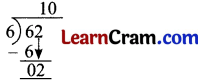Quotient = 10
Remainder = 2

(b) 508 ÷ 8
Solution: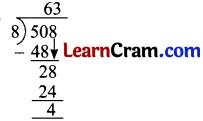Quotient = 63
Remainder = 4Question 5.
Seven boxes have 56 fire crackers. They are equally distributed in each box. How many fire crackers are there in one box?
Solution:
7 boxes have No. of fire crackers = 56
1 box has No. of fire crackers = 56 ÷ 7 = 8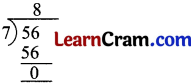1 box has 8 fire crackers

Question 6.
A man’s monthly salary is ₹ 9450. Find his yearly salary.
Solution:
1 year = 12 months
In 1 month man’s salary = ₹ 9450
In 12 months man’s salary = ₹ 9450 × 12
= ₹ 1,13,400Yearly salary is ₹ 1,13,400.Question 7.
Solve the puzzle.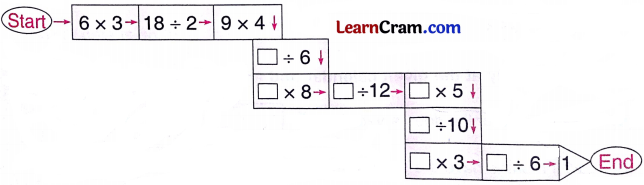Solution:Question 1.
Write the Division fact:
(a) 5 × 6 = 30
(b) 8 × 7 = 56
(c) 12 × 5 = 60
Solution: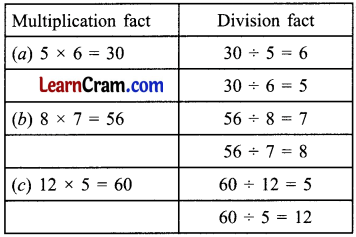Question 2.
Write the quotient and Remainder for the following.

(a) 90 ÷ 5
Solution:Quotient = 18
Remainder = 0

(b) 205 ÷ 5
Solution:Quotient = 41
Remainder = 0(c) 109 ÷ 3
Solution:Quotient = 36
Remainder = 1

(d) 200 ÷ 4
Solution: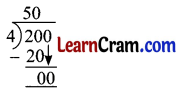Quotient = 50
Remainder = 0

(e) 220 ÷ 6
Solution: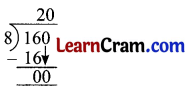Quotient = 36
Remainder = 4Question 3.
Division by Long division method.

(a) 2413 ÷ 3
Solution: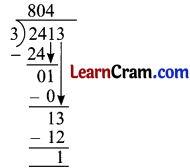Quotient = 804
Remainder = 1

(b) 570 ÷ 5
Solution: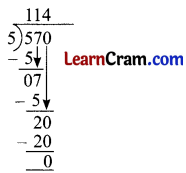Quotient = 114
Remainder = 0

(c) 364 ÷ 5
Solution: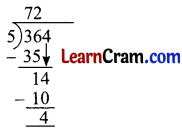Quotient = 72
Remainder = 1Question 4.
Find the pattern and fill in the box:

(a)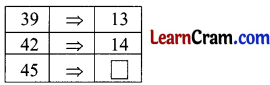Solution: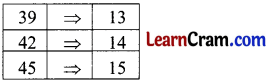(b)Solution:Question 5.
Using the properties of division solve the following:

(a) 17 ÷ 17 = ____
Solution:
17 ÷ 17 = 1

(b) 60 ÷ 60 = ____
Solution:
60 ÷ 60 = 1

(c) 0 ÷ 56 = ____
Solution:
0 ÷ 56 = 0

(d) 706 ÷ 1 = ____
Solution:
706 ÷ 1 = 706

(e) 17 ÷ 1 = ____
Solution:
17 ÷ 1 = 17